Updating search results...

# 33 Results

View
Selected filters:
• CCSS.Math.Content.8.EE.B.5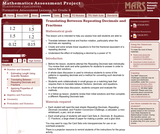Only Sharing Permitted
CC BY-NC-ND
Rating
4.0 stars

This lesson unit is intended to help teachers assess how well students are able to: translate between decimal and fraction notation, particularly when the decimals are repeating; create and solve simple linear equations to find the fractional equivalent of a repeating decimal; and understand the effect of multiplying a decimal by a power of 10.

Subject:
Algebra
Ratios and Proportions
Material Type:
Assessment
Lesson Plan
Provider:
Shell Center for Mathematical Education
Provider Set:
Mathematics Assessment Project (MAP)
04/26/2013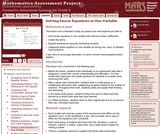Only Sharing Permitted
CC BY-NC-ND
Rating
5.0 stars

This lesson unit is intended to help teachers assess how well students are able to: solve linear equations in one variable with rational number coefficients; collect like terms; expand expressions using the distributive property; and categorize linear equations in one variable as having one, none, or infinitely many solutions. It also aims to encourage discussion on some common misconceptions about algebra.

Subject:
Algebra
Material Type:
Assessment
Lesson Plan
Provider:
Shell Center for Mathematical Education
Provider Set:
Mathematics Assessment Project (MAP)
04/26/2013Only Sharing Permitted
CC BY-NC-ND
Rating
4.0 stars

This lesson unit is intended to help teachers assess how well students are able to classify solutions to a pair of linear equations by considering their graphical representations. In particular, this unit aims to help teachers identify and assist students who have difficulties in: using substitution to complete a table of values for a linear equation; identifying a linear equation from a given table of values; and graphing and solving linear equations.

Subject:
Algebra
Material Type:
Assessment
Lesson Plan
Provider:
Shell Center for Mathematical Education
Provider Set:
Mathematics Assessment Project (MAP)
04/26/2013Educational Use
Rating
0.0 stars

In this video segment from Cyberchase, Harry decides to train as a firefighter and uses line graphs to chart his physical fitness progress.

Subject:
Mathematics
Physics
Material Type:
Lecture
Provider:
PBS LearningMedia
Provider Set:
PBS Learning Media: Multimedia Resources for the Classroom and Professional Development
Author:
U.S. Department of Education
WNET
09/25/2008Only Sharing Permitted
CC BY-NC-ND
Rating
5.0 stars

This lesson unit is intended to help teahcers assess how well students are able to interpret speed as the slope of a linear graph and translate between the equation of a line and its graphical representation.

Subject:
Algebra
Material Type:
Assessment
Lesson Plan
Provider:
Shell Center for Mathematical Education
Provider Set:
Mathematics Assessment Project (MAP)
04/26/2013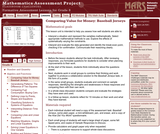Only Sharing Permitted
CC BY-NC-ND
Rating
4.0 stars

This lesson unit is intended to help teachers assess how well students are able to: interpret a situation and represent the variables mathematically; select appropriate mathematical methods to use; explore the effects of systematically varying the constraints; interpret and evaluate the data generated and identify the break-even point, checking it for confirmation; and communicate their reasoning clearly.

Subject:
Mathematics
Material Type:
Assessment
Lesson Plan
Provider:
Shell Center for Mathematical Education
Provider Set:
Mathematics Assessment Project (MAP)
04/26/2013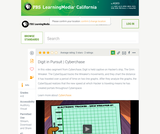Educational Use
Rating
0.0 stars

The CyberSquad tracks Digital position in time and then studies graphs to figure out what Hacker is scheming in this video from Cyberchase.

Subject:
Mathematics
Physics
Material Type:
Lecture
Provider:
PBS LearningMedia
Provider Set:
PBS Learning Media: Multimedia Resources for the Classroom and Professional Development
Author:
U.S. Department of Education
WNET
09/25/2008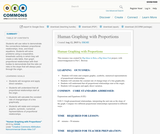Conditional Remix & Share Permitted
CC BY-NC-SA
Rating
0.0 stars

Students will use ratios to demonstrate the connections between proportional relationships, lines, and linear equations. Students will solve problems using a cooperative, kinesthetic activity in which they will create a ratio table, then graph proportional relationships with their bodies to demonstrate that the ratio (or rate) is the slope that will always pass through the origin.

Subject:
Mathematics
Material Type:
Activity/Lab
Teaching/Learning Strategy
Author:
01/28/2016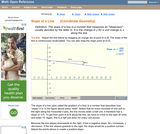Rating
0.0 stars

An interactive applet and associated web page that demonstrate the slope (m) of a line. The applet has two points that define a line. As the user drags either point it continuously recalculates the slope. The rise and run are drawn to show the two elements used in the calculation. The grid, axis pointers and coordinates can be turned on and off. The slope calculation can be turned off to permit class exercises and then turned back on the verify the answers. The applet can be printed as it appears on the screen to make handouts. The web page has a full description of the concept of slope, a worked example and has links to other pages relating to coordinate geometry. Applet can be enlarged to full screen size for use with a classroom projector. This resource is a component of the Math Open Reference Interactive Geometry textbook project at http://www.mathopenref.com.

Subject:
Geometry
Material Type:
Simulation
Provider:
Math Open Reference
Author:
John Page
02/16/2011Only Sharing Permitted
CC BY-NC-ND
Rating
3.0 stars

This lesson unit is intended to help teachers assess how well students are able to create and solve linear equations. In particular, the lesson will help you identify and help students who have the following difficulties: solving equations with one variable and solving linear equations in more than one way.

Subject:
Algebra
Material Type:
Assessment
Lesson Plan
Provider:
Shell Center for Mathematical Education
Provider Set:
Mathematics Assessment Project (MAP)
04/26/2013Only Sharing Permitted
CC BY-NC-ND
Rating
3.0 stars

This lesson unit is intended to help you assess how well students working with square numbers are able to: choose an appropriate, systematic way to collect and organize data, examining the data for patterns; describe and explain findings clearly and effectively; generalize using numerical, geometrical, graphical and/or algebraic structure; and explain why certain results are possible/impossible, moving towards a proof.

Subject:
Algebra
Geometry
Measurement and Data
Material Type:
Assessment
Lesson Plan
Provider:
Shell Center for Mathematical Education
Provider Set:
Mathematics Assessment Project (MAP)
04/26/2013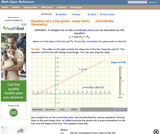Rating
0.0 stars

An interactive applet and associated web page that demonstrate the equation of a line in point-slope form. The user can move a slider that controls the slope, and can drag the point that defines the line. The graph changes accordingly and equation for the line is continuously recalculated with every slider and / or point move. The grid, axis pointers and coordinates can be turned on and off. The equation display can be turned off to permit class exercises and then turned back on the verify the answers. The applet can be printed as it appears on the screen to make handouts. The web page has a full description of the concept of the equation of a line in point - slope form, a worked example and has links to other pages relating to coordinate geometry. Applet can be enlarged to full screen size for use with a classroom projector. This resource is a component of the Math Open Reference Interactive Geometry textbook project at http://www.mathopenref.com.

Subject:
Geometry
Material Type:
Simulation
Provider:
Math Open Reference
Author:
John Page
02/16/2011
RemixConditional Remix & Share Permitted
CC BY-NC-SA
Rating
0.0 stars

Throughout this lesson, the teacher will explain equivalent fractions, plot them on a coordinate plane, connect and extend those points, then discuss the importance of the relationship and explore what the students notice about the relationship. This lesson can help depend understanding of equivalent fractions as well as deepen the discussion into slope being similar to the fraction that we gave at the beginning of the lesson. (Math Solutions Training idea)

Subject:
Mathematics
Material Type:
Activity/Lab
Author:
Cortney Ray
08/08/2019Unrestricted Use
CC BY
Rating
0.0 stars

This task requires students to work with unit rates.

Subject:
Mathematics
Material Type:
Activity/Lab
Provider:
Illustrative Mathematics
Provider Set:
Illustrative Mathematics
Author:
Illustrative Mathematics
05/01/2012Educational Use
Rating
0.0 stars

Students learn about the anatomy of the ear and how the ears work as a sound sensor. Ear anatomy parts and structures are explained in detail, as well as how sound is transmitted mechanically and then electrically through them to the brain. Students use LEGO® robots with sound sensors to measure sound intensities, learning how the NXT brick (computer) converts the intensity of sound measured by the sensor input into a number that transmits to a screen. They build on their experiences from the previous activities and establish a rich understanding of the sound sensor and its relationship to the TaskBot's computer.

Subject:
Engineering
Anatomy/Physiology
Material Type:
Activity/Lab
Provider:
TeachEngineering
Provider Set:
TeachEngineering
Author:
Charlie Franklin
Marianne Catanho
Sachin Nair
Satish Nair
09/18/2014Only Sharing Permitted
CC BY-NC-ND
Rating
4.0 stars

This lesson unit is intended to help teachers assess how well students are able to: estimate lengths of everyday objects; convert between decimal and scientific notation; and make comparisons of the size of numbers expressed in both decimal and scientific notation.

Subject:
Mathematics
Material Type:
Assessment
Lesson Plan
Provider:
Shell Center for Mathematical Education
Provider Set:
Mathematics Assessment Project (MAP)
04/26/2013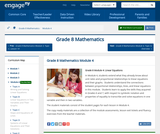Conditional Remix & Share Permitted
CC BY-NC-SA
Rating
0.0 stars

In Module 4, students extend what they already know about unit rates and proportional relationships to linear equations and their graphs.  Students understand the connections between proportional relationships, lines, and linear equations in this module.  Students learn to apply the skills they acquired in Grades 6 and 7, with respect to symbolic notation and properties of equality to transcribe and solve equations in one variable and then in two variables.

Subject:
Algebra
Material Type:
Module
Provider:
New York State Education Department
Provider Set:
EngageNY
11/12/2013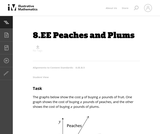Unrestricted Use
CC BY
Rating
0.0 stars

This task allows students to reason about the relative costs per pound of the two fruits without actually knowing what the costs are. Students who find this difficult may add a scale to the graph and reason about the meanings of the ordered pairs. Comparing the two approaches in a class discussion can be a profitable way to help students make sense of slope.

Subject:
Mathematics
Material Type:
Activity/Lab
Provider:
Illustrative Mathematics
Provider Set:
Illustrative Mathematics
Author:
Illustrative Mathematics
05/01/2012Unrestricted Use
CC BY
Rating
0.0 stars

This is a task from the Illustrative Mathematics website that is one part of a complete illustration of the standard to which it is aligned. Each task has at least one solution and some commentary that addresses important asects of the task and its potential use. Here are the first few lines of the commentary for this task: Anna and Jason have summer jobs stuffing envelopes for two different companies. Anna earns \\$14 for every 400 envelops she finishes. Jason earns \\$9 fo...

Subject:
Mathematics
Material Type:
Activity/Lab
Provider:
Illustrative Mathematics
Provider Set:
Illustrative Mathematics
Author:
Illustrative Mathematics
08/12/2013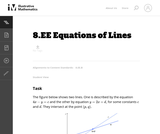Unrestricted Use
CC BY
Rating
0.0 stars

This task requires students to use the fact that on the graph of the linear equation y=ax+c, the y-coordinate increases by a when x increases by one. Specific values for c and d were left out intentionally to encourage students to use the above fact as opposed to computing the point of intersection, (p,q), and then computing respective function values to answer the question.

Subject:
Mathematics
Material Type:
Activity/Lab
Provider:
Illustrative Mathematics
Provider Set:
Illustrative Mathematics
Author:
Illustrative Mathematics